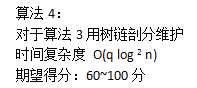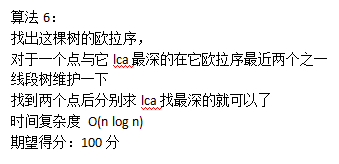# DFS 序和欧拉序复习

29 October 2019 | duanyll | Tags: OI 图论

## 一道题

• 0操作: 将一颗棋子翻转
• 1操作:询问一颗棋子与所有面朝上为黑色的棋子lca最深的那个的编号## DFS 序

1. 一个节点和它的子树在一个连续的区间中.
2. 进入时间是 dfs 序的反函数, 即 dfs 序中的第i个点的进入时间是i.

## 应用

### 树链修改, 单点查询

1. x 到根节点的链上所有节点权值加 v.
2. y 到根节点的链上所有节点权值加 v.
3. lca(x, y) 到根节点的链上所有节点权值减 v.
4. fa[lca(x, y)] 到根节点的链上所有节点权值减 v.

update(l[x], v);
update(l[y], v);
update(l[lca(x, y)], -v);
update(l[fa(lca(x, y))], -v)


sum(r[x]) - sum(l[x] - 1)


### 单点更新, 树链和查询

update(l[x], v);
update(r[x] + 1, -v)


sum(l[x]) + sum(l[y]) - sum(l[lca(x, y)]) - sum(l[fa(lca(x, y))])


### 其他

• 子树修改, 单点查询: 区间修改, 单点查询.
• 子树修改, 子树和查询: 区间修改, 区间查询
• 子树修改, 树链查询: 定义 $w[u]$ 为根节点到y节点的链上所有节点和, 查询和修改操作类似可推.